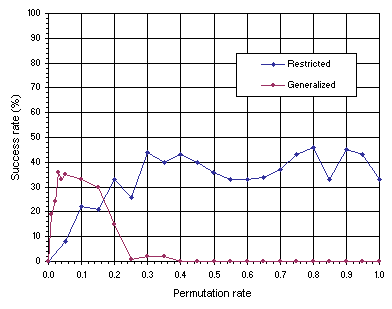Home
News
Author
Q&A
Tutorials
GEP Biblio
Contacts

Visit Gepsoft

 Generalized permutation is a variation of the restricted permutation operator described in section 6.2.3. Recall that during restricted permutation only a pair of genes are exchanged per chromosome, that is, the restricted permutation rate prp is evaluated by prp = NC / P, where NC represents the number of chromosomes modified. A more generalized version of this operator can be easily implemented where a different number of genes in a chromosome can trade places with other genes according to a certain rate. More formally, the generalized permutation rate pgp is evaluated by pgp = NG / (CL x P), where NG represents the number of genes modified and CL the chromosome length. Again, this might appear more efficient than the restricted permutation described in section 6.2.3, but experience shows that restricted permutation is slightly better (see Figure 6.4 below). For instance, in the TSP with 19 cities (see Figure 6.2), generalized permutation performed worse than restricted permutation and, in fact, was incapable of finding a perfect solution. The results obtained for a simpler version of the TSP with 13 cities are shown in Figure 6.4. In this analysis, both the restricted permutation and generalized permutation operators are compared using populations of 100 individuals evolving for 200 generations, i.e., exactly the same values of P and G used to solve the much more complex TSP with 19 cities shown in Figure 6.2.Figure 6.4. Comparison of restricted permutation with generalized permutation on the traveling salesperson problem with 13 cities. For this analysis P = 100 and G = 200. The success rate was evaluated over 100 identical runs.